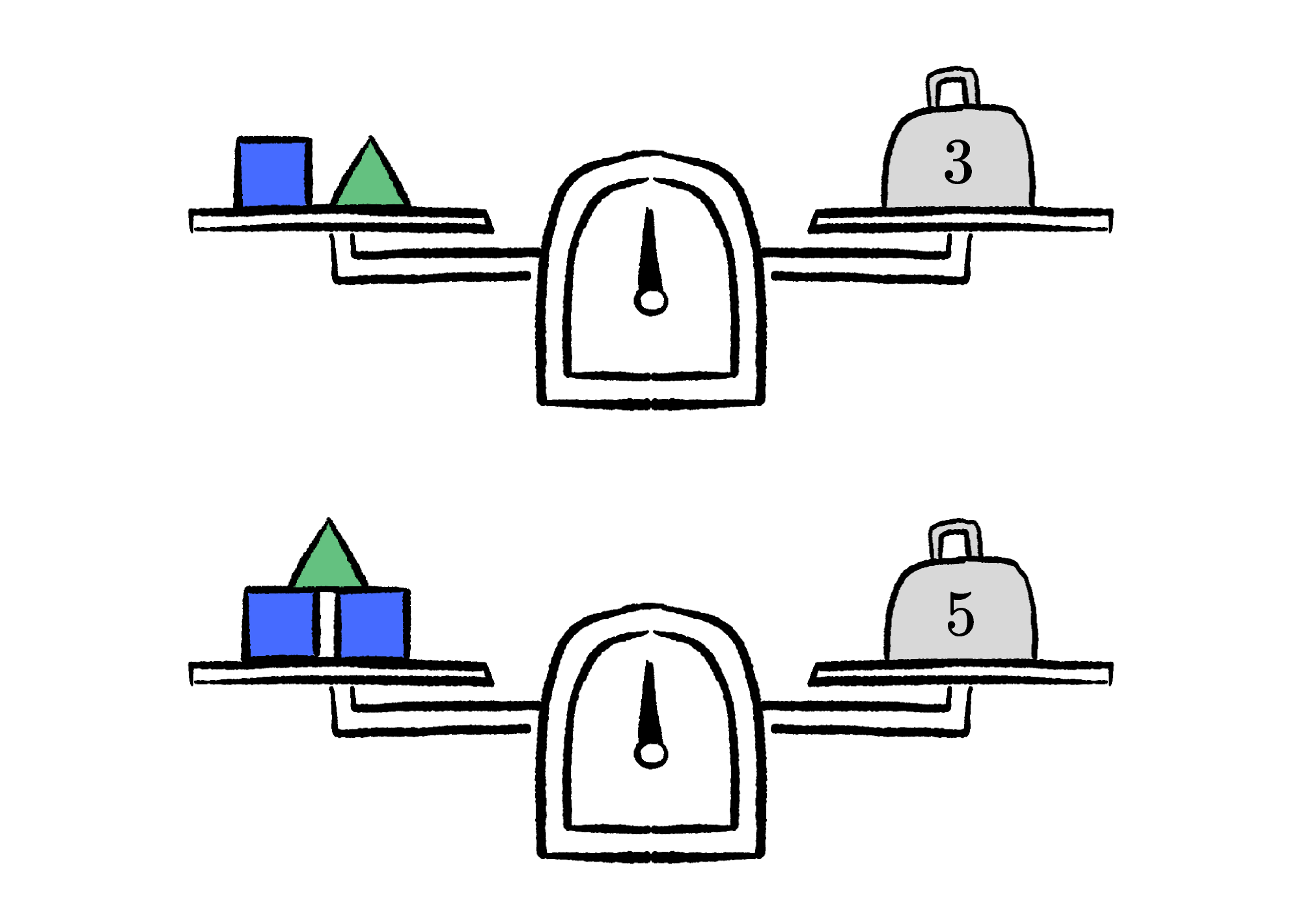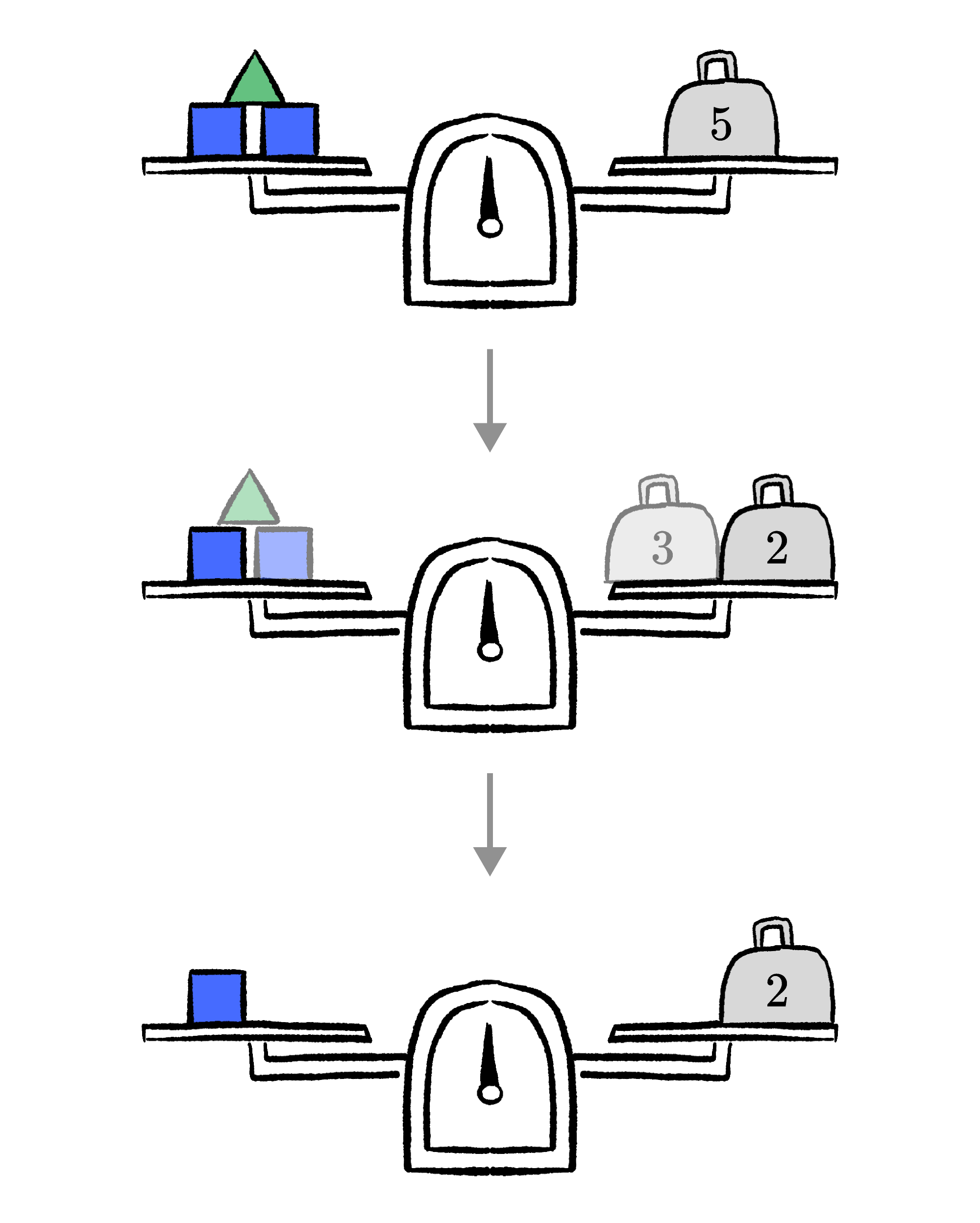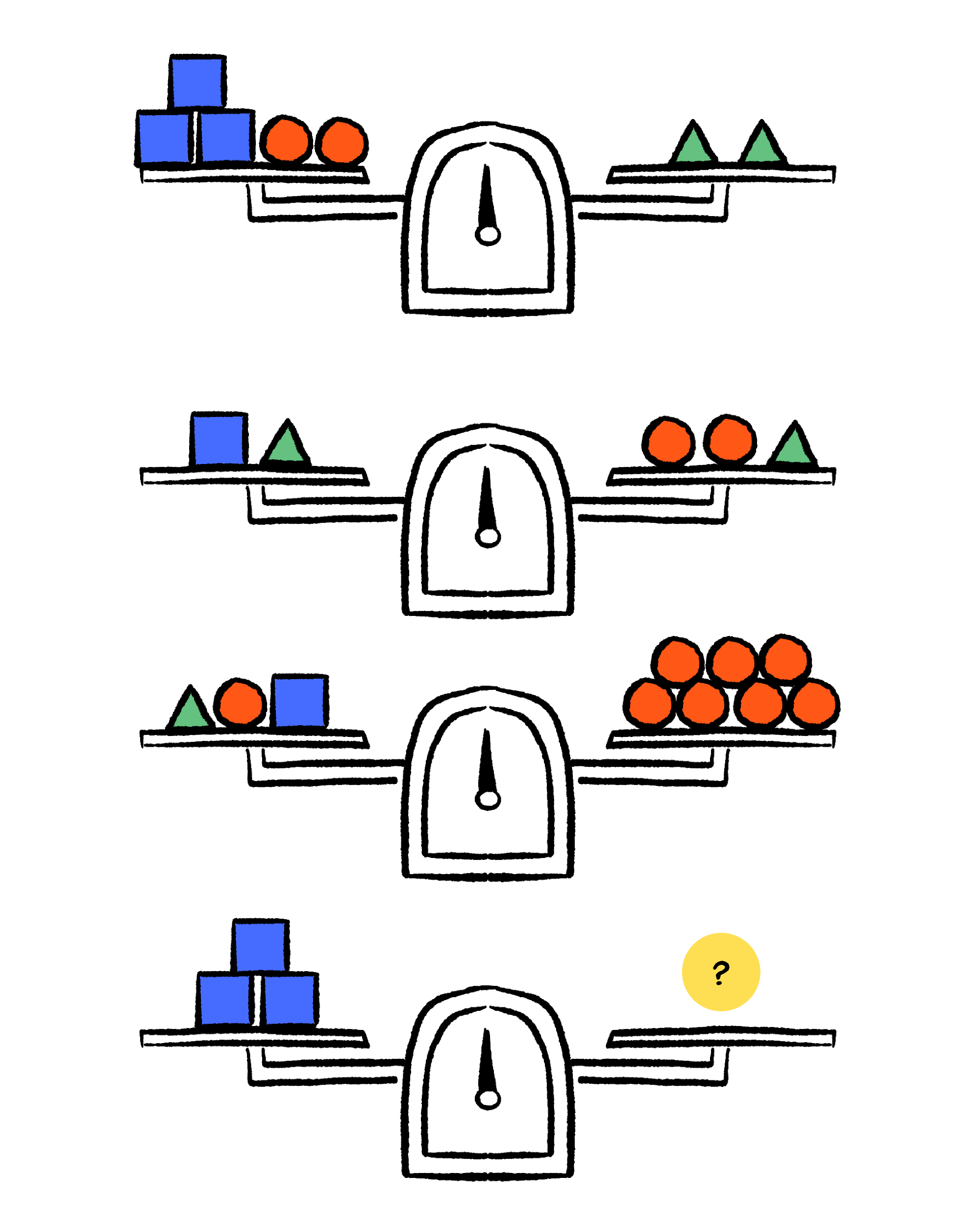Back

## Stabilize These Squares

Given the two balance scales, we might ask how much the green triangle weighs.Or perhaps, we might want to know the value of $t$ in the equations \begin{aligned} s + t &= 3 \\ 2s + t &= 5. \end{aligned} In either case, we can use the same approach: the elimination method.

Elimination depends on this fact:

We can always keep a scale in balance by removing (or adding) equivalent quantities on both sides.What's happening here? We know from the first balance that a square and a triangle are equivalent to $3$ (they are in balance and must therefore weigh the same). Therefore, on the second balance, we can remove a square and a triangle from the left and $3$ from the right, and the scale will still be in balance.

Note that although what we removed on the right looks different from what we removed on the left, we know that they are the equivalent because the first scale showed us.

From here, we can solve directly: the blue square equals $2,$ so the green triangle must equal $1.$Let's look at the same approach but this time with an algebraic representation: \begin{aligned} s + t &= 3 \\ 2s + t &= 5. \end{aligned} Subtracting the first equation from the second, we get \begin{aligned} 2s + t &= 5 \\ - (s + t) &= -(3) \\ \Rightarrow s &= 2. \end{aligned} Because $s=2$, we can conclude that $t=1$. By combining these equations, we found the solution very quickly.

Although the problem below looks cumbersome, if you approach it as an elimination problem — where combining the equations together lets you simplify — there's a straightforward path to the solution.

# Today's Challenge

Given the balance scales below, which combination would balance with three squares?×